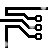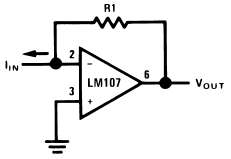## The Current-to-Voltage Converter

### By internum

The current-to-voltage transducer.

Current may be measured in two ways with an operational amplifier. The current may be converted into a voltage with a resistor and then amplified or the current may be injected directly into a summing node. Converting into voltage is undesirable for two reasons: first, an impedance is inserted into the measuring line causing an error; second, amplifier offset voltage is also amplified with a subsequent loss of accuracy. The use of a current-to-voltage transducer avoids both of these problems.Figure 1. Current to Voltage ConverterThe current-to-voltage transducer is shown in Figure 1. The input current is fed directly into the summing node and the amplifier output voltage changes to extract the same current from the summing node through R1. The scale factor of this circuit is R1 volts per amp. The only conversion error in this circuit is Ibias which is summed algebraically with IIN.

This basic circuit is useful for many applications other than current measurement. It is shown as a photocell amplifier in the following section.

The only design constraints are that scale factors must be chosen to minimize errors due to bias current and since voltage gain and source impedance are often indeterminate (as with photocells) the amplifier must be compensated for unity-gain operation.130909 December 2007